8 Potential Energy and Conservation of Energy

8.2 Conservative and Non-Conservative Forces

Learning Objectives

By the end of this section, you will be able to:

• Characterize a conservative force in several different ways
• Specify mathematical conditions that must be satisfied by a conservative force and its components
• Relate the conservative force between particles of a system to the potential energy of the system
• Calculate the components of a conservative force in various cases

In Potential Energy and Conservation of Energy, any transition between kinetic and potential energy conserved the total energy of the system. This was path independent, meaning that we can start and stop at any two points in the problem, and the total energy of the system—kinetic plus potential—at these points are equal to each other. This is characteristic of a conservative force. We dealt with conservative forces in the preceding section, such as the gravitational force and spring force. When comparing the motion of the football in (Figure), the total energy of the system never changes, even though the gravitational potential energy of the football increases, as the ball rises relative to ground and falls back to the initial gravitational potential energy when the football player catches the ball. Non-conservative forces are dissipative forces such as friction or air resistance. These forces take energy away from the system as the system progresses, energy that you can’t get back. These forces are path dependent; therefore it matters where the object starts and stops.

Conservative Force

The work done by a conservative force is independent of the path; in other words, the work done by a conservative force is the same for any path connecting two points: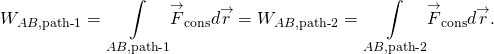The work done by a non-conservative force depends on the path taken.

Equivalently, a force is conservative if the work it does around any closed path is zero: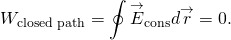[In (Figure), we use the notation of a circle in the middle of the integral sign for a line integral over a closed path, a notation found in most physics and engineering texts.] (Figure) and (Figure) are equivalent because any closed path is the sum of two paths: the first going from A to B, and the second going from B to A. The work done going along a path from B to A is the negative of the work done going along the same path from A to B, where A and B are any two points on the closed path: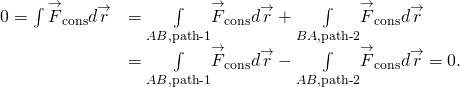You might ask how we go about proving whether or not a force is conservative, since the definitions involve any and all paths from A to B, or any and all closed paths, but to do the integral for the work, you have to choose a particular path. One answer is that the work done is independent of path if the infinitesimal work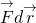is an exact differential, the way the infinitesimal net work was equal to the exact differential of the kinetic energy,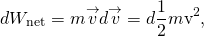when we derived the work-energy theorem in Work-Energy Theorem. There are mathematical conditions that you can use to test whether the infinitesimal work done by a force is an exact differential, and the force is conservative. These conditions only involve differentiation and are thus relatively easy to apply. In two dimensions, the condition for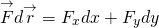to be an exact differential is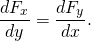You may recall that the work done by the force in (Figure) depended on the path. For that force,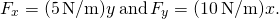Therefore,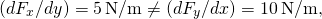which indicates it is a non-conservative force. Can you see what you could change to make it a conservative force?Figure 8.5 A grinding wheel applies a non-conservative force, because the work done depends on how many rotations the wheel makes, so it is path-dependent.

Example

Conservative or Not?

Which of the following two-dimensional forces are conservative and which are not? Assume a and b are constants with appropriate units:

(a)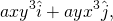(b)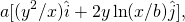(c)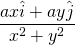Strategy

Apply the condition stated in (Figure), namely, using the derivatives of the components of each force indicated. If the derivative of the y-component of the force with respect to x is equal to the derivative of the x-component of the force with respect to y, the force is a conservative force, which means the path taken for potential energy or work calculations always yields the same results.

Solution

1.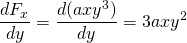and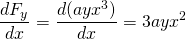, so this force is non-conservative.

2.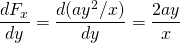and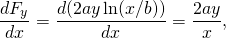so this force is conservative.

3.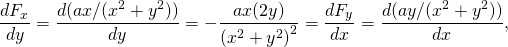again conservative.

Significance

The conditions in (Figure) are derivatives as functions of a single variable; in three dimensions, similar conditions exist that involve more derivatives.

A two-dimensional, conservative force is zero on the x– and y-axes, and satisfies the condition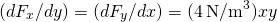. What is the magnitude of the force at the point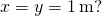2.83 N

Before leaving this section, we note that non-conservative forces do not have potential energy associated with them because the energy is lost to the system and can’t be turned into useful work later. So there is always a conservative force associated with every potential energy. We have seen that potential energy is defined in relation to the work done by conservative forces. That relation, (Figure), involved an integral for the work; starting with the force and displacement, you integrated to get the work and the change in potential energy. However, integration is the inverse operation of differentiation; you could equally well have started with the potential energy and taken its derivative, with respect to displacement, to get the force. The infinitesimal increment of potential energy is the dot product of the force and the infinitesimal displacement,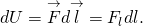Here, we chose to represent the displacement in an arbitrary direction by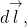so as not to be restricted to any particular coordinate direction. We also expressed the dot product in terms of the magnitude of the infinitesimal displacement and the component of the force in its direction. Both these quantities are scalars, so you can divide by dl to get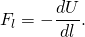This equation gives the relation between force and the potential energy associated with it. In words, the component of a conservative force, in a particular direction, equals the negative of the derivative of the corresponding potential energy, with respect to a displacement in that direction. For one-dimensional motion, say along the x-axis, (Figure) give the entire vector force,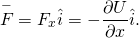In two dimensions,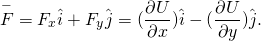From this equation, you can see why (Figure) is the condition for the work to be an exact differential, in terms of the derivatives of the components of the force. In general, a partial derivative notation is used. If a function has many variables in it, the derivative is taken only of the variable the partial derivative specifies. The other variables are held constant. In three dimensions, you add another term for the z-component, and the result is that the force is the negative of the gradient of the potential energy. However, we won’t be looking at three-dimensional examples just yet.

Example

Force due to a Quartic Potential Energy

The potential energy for a particle undergoing one-dimensional motion along the x-axis is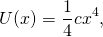whereIts total energy at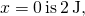and it is not subject to any non-conservative forces. Find (a) the positions where its kinetic energy is zero and (b) the forces at those positions.

Strategy

(a) We can find the positions where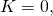so the potential energy equals the total energy of the given system. (b) Using (Figure), we can find the force evaluated at the positions found from the previous part, since the mechanical energy is conserved.

Solution

1. The total energy of the system of 2 J equals the quartic elastic energy as given in the problem,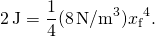Solving for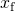results in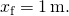2. From (Figure),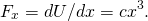Thus, evaluating the force at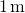, we get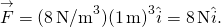At both positions, the magnitude of the forces is 8 N and the directions are toward the origin, since this is the potential energy for a restoring force.

Significance

Finding the force from the potential energy is mathematically easier than finding the potential energy from the force, because differentiating a function is generally easier than integrating one.

Find the forces on the particle in (Figure) when its kinetic energy is 1.0 J at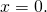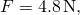directed toward the origin

Summary

• A conservative force is one for which the work done is independent of path. Equivalently, a force is conservative if the work done over any closed path is zero.
• A non-conservative force is one for which the work done depends on the path.
• For a conservative force, the infinitesimal work is an exact differential. This implies conditions on the derivatives of the force’s components.
• The component of a conservative force, in a particular direction, equals the negative of the derivative of the potential energy for that force, with respect to a displacement in that direction.

Conceptual Questions

What is the physical meaning of a non-conservative force?

A force that takes energy away from the system that can’t be recovered if we were to reverse the action.

A bottle rocket is shot straight up in the air with a speed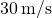. If the air resistance is ignored, the bottle would go up to a height of approximately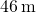. However, the rocket goes up to only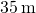before returning to the ground. What happened? Explain, giving only a qualitative response.

An external force acts on a particle during a trip from one point to another and back to that same point. This particle is only effected by conservative forces. Does this particle’s kinetic energy and potential energy change as a result of this trip?

The change in kinetic energy is the net work. Since conservative forces are path independent, when you are back to the same point the kinetic and potential energies are exactly the same as the beginning. During the trip the total energy is conserved, but both the potential and kinetic energy change.

Problems

A force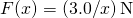acts on a particle as it moves along the positive x-axis. (a) How much work does the force do on the particle as it moves from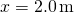to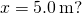(b) Picking a convenient reference point of the potential energy to be zero at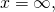find the potential energy for this force.

A force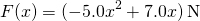acts on a particle. (a) How much work does the force do on the particle as it moves fromto(b) Picking a convenient reference point of the potential energy to be zero atfind the potential energy for this force.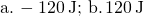Find the force corresponding to the potential energy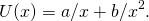The potential energy function for either one of the two atoms in a diatomic molecule is often approximated by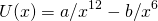where x is the distance between the atoms. (a) At what distance of seperation does the potential energy have a local minimum (not at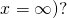(b) What is the force on an atom at this separation? (c) How does the force vary with the separation distance?

a.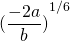; b.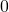; c.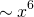A particle of mass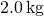moves under the influence of the force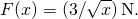If its speed atis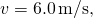what is its speed at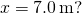A particle of massmoves under the influence of the force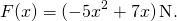If its speed at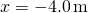is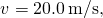what is its speed at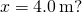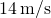A crate on rollers is being pushed without frictional loss of energy across the floor of a freight car (see the following figure). The car is moving to the right with a constant speed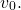If the crate starts at rest relative to the freight car, then from the work-energy theorem,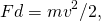where d, the distance the crate moves, and v, the speed of the crate, are both measured relative to the freight car. (a) To an observer at rest beside the tracks, what distance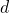is the crate pushed when it moves the distance d in the car? (b) What are the crate’s initial and final speeds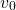and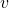as measured by the observer beside the tracks? (c) Show that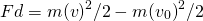and, consequently, that work is equal to the change in kinetic energy in both reference systems.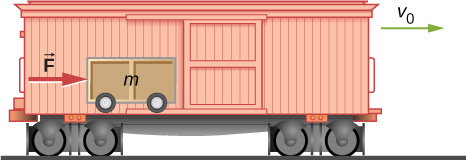Glossary

conservative force
force that does work independent of path
exact differential
is the total differential of a function and requires the use of partial derivatives if the function involves more than one dimension
non-conservative force
force that does work that depends on path